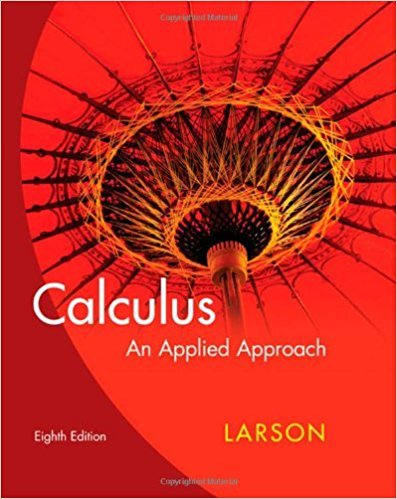×
×

# Solutions for Chapter 2.2: Some Rules for Differentiation## Full solutions for Calculus: An Applied Approach | 8th Edition

ISBN: 9780618958252Solutions for Chapter 2.2: Some Rules for Differentiation

Solutions for Chapter 2.2
4 5 0 345 Reviews
11
3
##### ISBN: 9780618958252

Chapter 2.2: Some Rules for Differentiation includes 68 full step-by-step solutions. Calculus: An Applied Approach was written by and is associated to the ISBN: 9780618958252. This textbook survival guide was created for the textbook: Calculus: An Applied Approach , edition: 8. This expansive textbook survival guide covers the following chapters and their solutions. Since 68 problems in chapter 2.2: Some Rules for Differentiation have been answered, more than 24093 students have viewed full step-by-step solutions from this chapter.

Key Calculus Terms and definitions covered in this textbook
• Bounded interval

An interval that has finite length (does not extend to ? or -?)

• Boxplot (or box-and-whisker plot)

A graph that displays a five-number summary

• Center

The central point in a circle, ellipse, hyperbola, or sphere

• Component form of a vector

If a vector’s representative in standard position has a terminal point (a,b) (or (a, b, c)) , then (a,b) (or (a, b, c)) is the component form of the vector, and a and b are the horizontal and vertical components of the vector (or a, b, and c are the x-, y-, and z-components of the vector, respectively)

• Compound fraction

A fractional expression in which the numerator or denominator may contain fractions

• Cosine

The function y = cos x

• Coterminal angles

Two angles having the same initial side and the same terminal side

• Data

Facts collected for statistical purposes (singular form is datum)

• Degree of a polynomial (function)

The largest exponent on the variable in any of the terms of the polynomial (function)

• Derivative of ƒ at x a

ƒ'(a) = lim x:a ƒ(x) - ƒ(a) x - a provided the limit exists

• equation of a parabola

(x - h)2 = 4p(y - k) or (y - k)2 = 4p(x - h)

• equation of a quadratic function

ƒ(x) = ax 2 + bx + c(a ? 0)

• equation of an ellipse

(x - h2) a2 + (y - k)2 b2 = 1 or (y - k)2 a2 + (x - h)2 b2 = 1

• Horizontal line

y = b.

• Local extremum

A local maximum or a local minimum

• Magnitude of a vector

The magnitude of <a, b> is 2a2 + b2. The magnitude of <a, b, c> is 2a2 + b2 + c2

• Transitive property

If a = b and b = c , then a = c. Similar properties hold for the inequality symbols <, >, ?, ?.

• Unit circle

A circle with radius 1 centered at the origin.

• Venn diagram

A visualization of the relationships among events within a sample space.

• Window dimensions

The restrictions on x and y that specify a viewing window. See Viewing window.

×